# GA.pptx

5 Sep 2022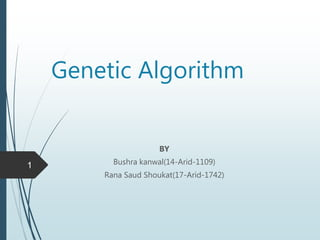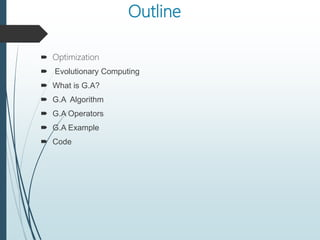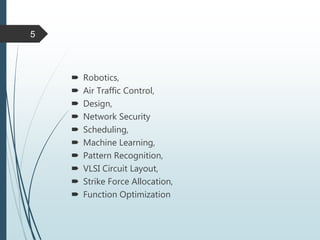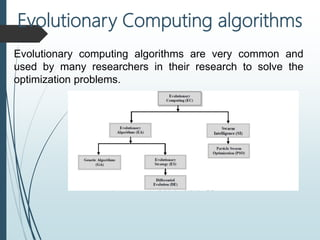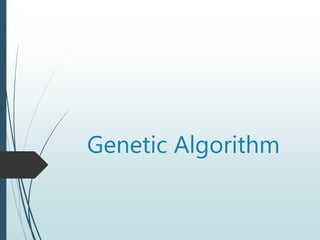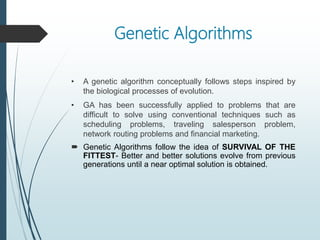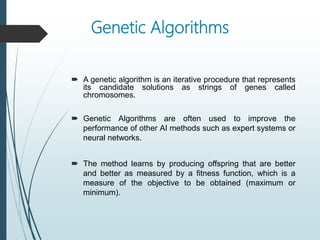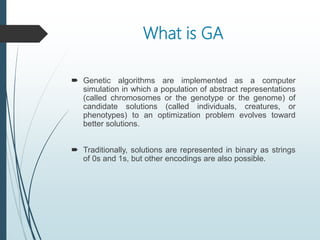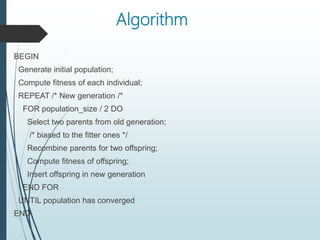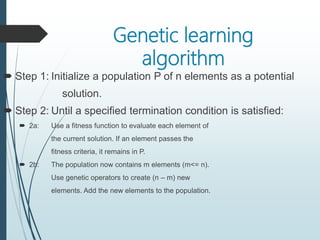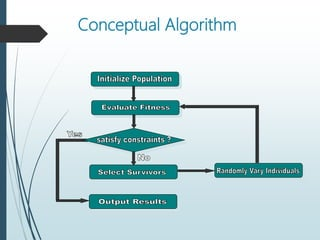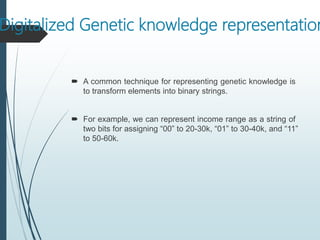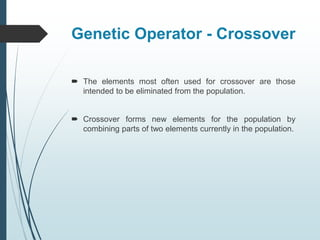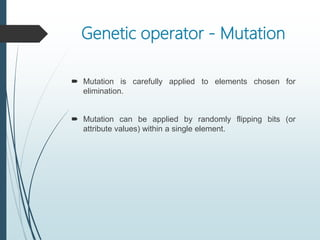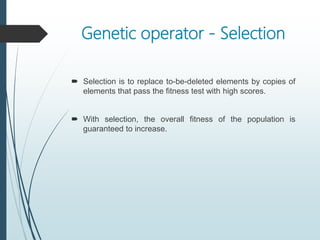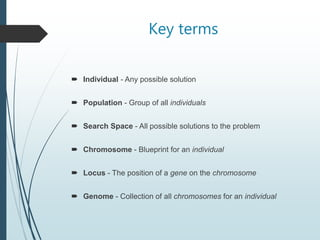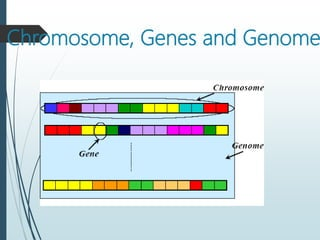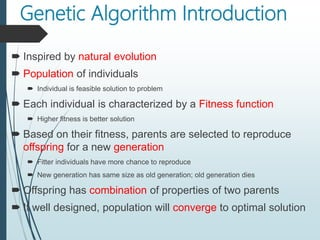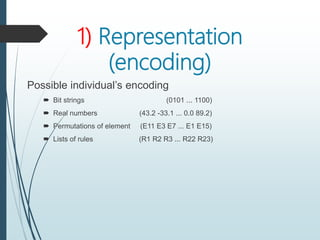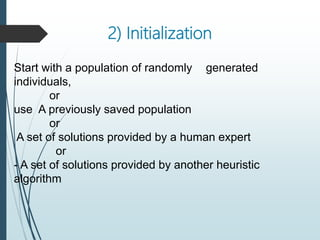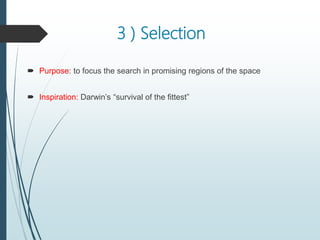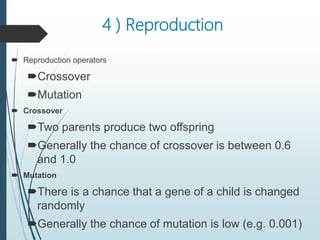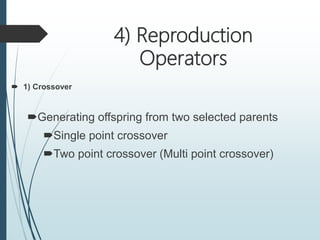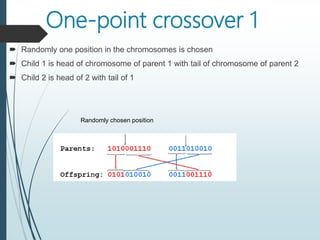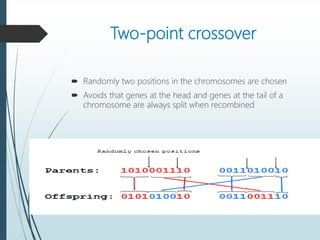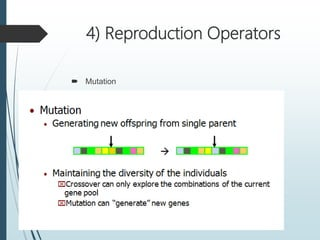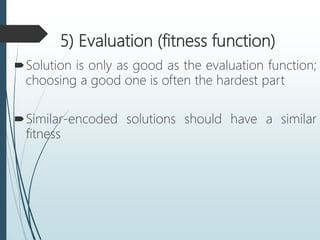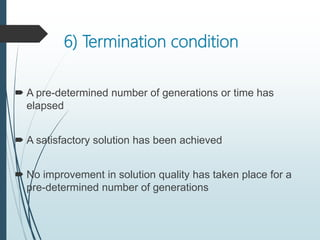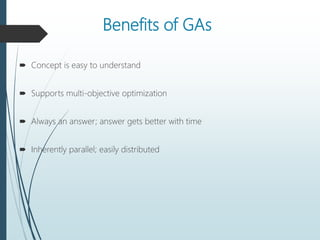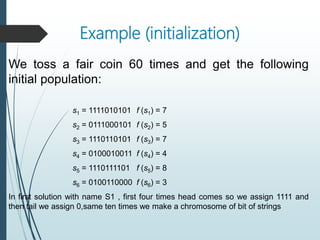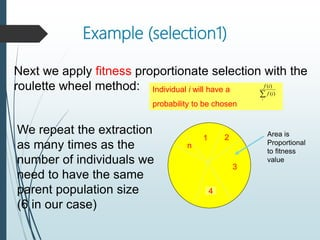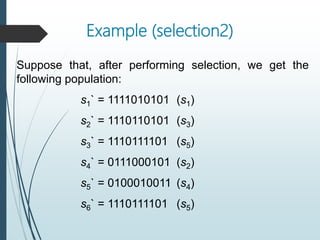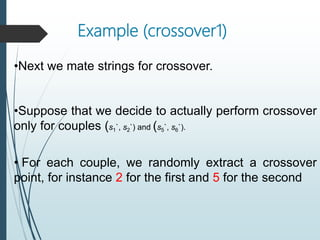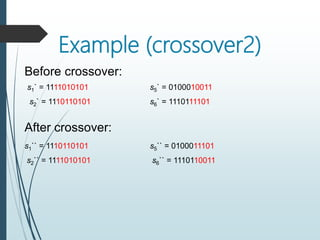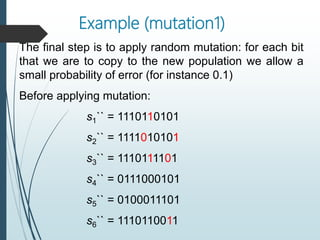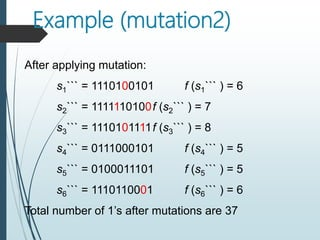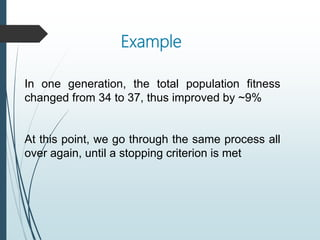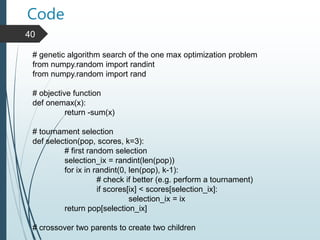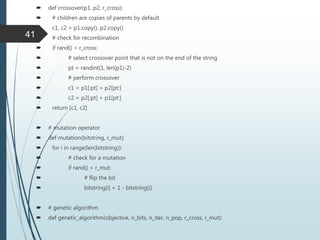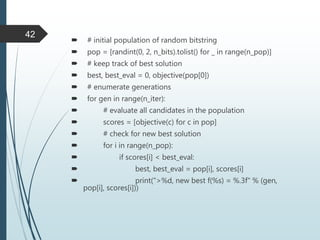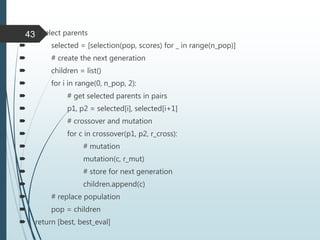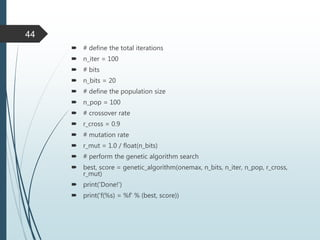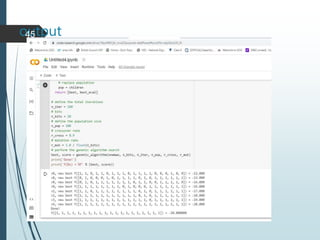1 sur 44

### GA.pptx

• 2. Outline  Optimization  Evolutionary Computing  What is G.A?  G.A Algorithm  G.A Operators  G.A Example  Code
• 3. Evolutionary Computation  Evolutionary Computation is the field of study devoted to the design, development, and analysis is problem solvers based on natural selection (simulated evolution).  Evolution has proven to be a powerful search process.  Evolutionary Computation has been successfully applied to a wide range of problems including:  Aircraft Design,  Routing in Communications Networks,  Tracking Windshear,  Game Playing (Checkers [Fogel]) 4
• 4.  Robotics,  Air Traffic Control,  Design,  Network Security  Scheduling,  Machine Learning,  Pattern Recognition,  VLSI Circuit Layout,  Strike Force Allocation,  Function Optimization 5
• 5. Evolutionary computing algorithms are very common and used by many researchers in their research to solve the optimization problems. Evolutionary Computing algorithms
• 7. Genetic Algorithms • A genetic algorithm conceptually follows steps inspired by the biological processes of evolution. • GA has been successfully applied to problems that are difficult to solve using conventional techniques such as scheduling problems, traveling salesperson problem, network routing problems and financial marketing.  Genetic Algorithms follow the idea of SURVIVAL OF THE FITTEST- Better and better solutions evolve from previous generations until a near optimal solution is obtained.
• 8. Genetic Algorithms  A genetic algorithm is an iterative procedure that represents its candidate solutions as strings of genes called chromosomes.  Genetic Algorithms are often used to improve the performance of other AI methods such as expert systems or neural networks.  The method learns by producing offspring that are better and better as measured by a fitness function, which is a measure of the objective to be obtained (maximum or minimum).
• 9. What is GA  Genetic algorithms are implemented as a computer simulation in which a population of abstract representations (called chromosomes or the genotype or the genome) of candidate solutions (called individuals, creatures, or phenotypes) to an optimization problem evolves toward better solutions.  Traditionally, solutions are represented in binary as strings of 0s and 1s, but other encodings are also possible.
• 10. Algorithm BEGIN Generate initial population; Compute fitness of each individual; REPEAT /* New generation /* FOR population_size / 2 DO Select two parents from old generation; /* biased to the fitter ones */ Recombine parents for two offspring; Compute fitness of offspring; Insert offspring in new generation END FOR UNTIL population has converged END
• 11. Genetic learning algorithm  Step 1: Initialize a population P of n elements as a potential solution.  Step 2: Until a specified termination condition is satisfied:  2a: Use a fitness function to evaluate each element of the current solution. If an element passes the fitness criteria, it remains in P.  2b: The population now contains m elements (m<= n). Use genetic operators to create (n – m) new elements. Add the new elements to the population.
• 13. Digitalized Genetic knowledge representation  A common technique for representing genetic knowledge is to transform elements into binary strings.  For example, we can represent income range as a string of two bits for assigning “00” to 20-30k, “01” to 30-40k, and “11” to 50-60k.
• 14. Genetic Operator - Crossover  The elements most often used for crossover are those intended to be eliminated from the population.  Crossover forms new elements for the population by combining parts of two elements currently in the population.
• 15. Genetic operator - Mutation  Mutation is carefully applied to elements chosen for elimination.  Mutation can be applied by randomly flipping bits (or attribute values) within a single element.
• 16. Genetic operator - Selection  Selection is to replace to-be-deleted elements by copies of elements that pass the fitness test with high scores.  With selection, the overall fitness of the population is guaranteed to increase.
• 17. Key terms  Individual - Any possible solution  Population - Group of all individuals  Search Space - All possible solutions to the problem  Chromosome - Blueprint for an individual  Locus - The position of a gene on the chromosome  Genome - Collection of all chromosomes for an individual
• 19. Genetic Algorithm Introduction  Inspired by natural evolution  Population of individuals  Individual is feasible solution to problem  Each individual is characterized by a Fitness function  Higher fitness is better solution  Based on their fitness, parents are selected to reproduce offspring for a new generation  Fitter individuals have more chance to reproduce  New generation has same size as old generation; old generation dies  Offspring has combination of properties of two parents  If well designed, population will converge to optimal solution
• 20. 1) Representation (encoding) Possible individual’s encoding  Bit strings (0101 ... 1100)  Real numbers (43.2 -33.1 ... 0.0 89.2)  Permutations of element (E11 E3 E7 ... E1 E15)  Lists of rules (R1 R2 R3 ... R22 R23)
• 21. 2) Initialization Start with a population of randomly generated individuals, or use A previously saved population or A set of solutions provided by a human expert or - A set of solutions provided by another heuristic algorithm
• 22. 3 ) Selection  Purpose: to focus the search in promising regions of the space  Inspiration: Darwin’s “survival of the fittest”
• 23. 4 ) Reproduction  Reproduction operators Crossover Mutation  Crossover Two parents produce two offspring Generally the chance of crossover is between 0.6 and 1.0  Mutation There is a chance that a gene of a child is changed randomly Generally the chance of mutation is low (e.g. 0.001)
• 24. 4) Reproduction Operators  1) Crossover Generating offspring from two selected parents Single point crossover Two point crossover (Multi point crossover)
• 25. One-point crossover 1  Randomly one position in the chromosomes is chosen  Child 1 is head of chromosome of parent 1 with tail of chromosome of parent 2  Child 2 is head of 2 with tail of 1 Randomly chosen position
• 26. Two-point crossover  Randomly two positions in the chromosomes are chosen  Avoids that genes at the head and genes at the tail of a chromosome are always split when recombined
• 28. 5) Evaluation (fitness function) Solution is only as good as the evaluation function; choosing a good one is often the hardest part Similar-encoded solutions should have a similar fitness
• 29. 6) Termination condition  A pre-determined number of generations or time has elapsed  A satisfactory solution has been achieved  No improvement in solution quality has taken place for a pre-determined number of generations
• 30. Benefits of GAs  Concept is easy to understand  Supports multi-objective optimization  Always an answer; answer gets better with time  Inherently parallel; easily distributed
• 31. Example (initialization) We toss a fair coin 60 times and get the following initial population: s1 = 1111010101 f (s1) = 7 s2 = 0111000101 f (s2) = 5 s3 = 1110110101 f (s3) = 7 s4 = 0100010011 f (s4) = 4 s5 = 1110111101 f (s5) = 8 s6 = 0100110000 f (s6) = 3 In first solution with name S1 , first four times head comes so we assign 1111 and then tail we assign 0,same ten times we make a chromosome of bit of strings
• 32. Example (selection1) Next we apply fitness proportionate selection with the roulette wheel method: 2 1 n 3 Area is Proportional to fitness value Individual i will have a probability to be chosen  i i f i f ) ( ) ( 4 We repeat the extraction as many times as the number of individuals we need to have the same parent population size (6 in our case)
• 33. Example (selection2) Suppose that, after performing selection, we get the following population: s1` = 1111010101 (s1) s2` = 1110110101 (s3) s3` = 1110111101 (s5) s4` = 0111000101 (s2) s5` = 0100010011 (s4) s6` = 1110111101 (s5)
• 34. Example (crossover1) •Next we mate strings for crossover. •Suppose that we decide to actually perform crossover only for couples (s1`, s2`) and (s5`, s6`). • For each couple, we randomly extract a crossover point, for instance 2 for the first and 5 for the second
• 35. Example (crossover2) s1` = 1111010101 s2` = 1110110101 s5` = 0100010011 s6` = 1110111101 Before crossover: After crossover: s1`` = 1110110101 s2`` = 1111010101 s5`` = 0100011101 s6`` = 1110110011
• 36. Example (mutation1) The final step is to apply random mutation: for each bit that we are to copy to the new population we allow a small probability of error (for instance 0.1) Before applying mutation: s1`` = 1110110101 s2`` = 1111010101 s3`` = 1110111101 s4`` = 0111000101 s5`` = 0100011101 s6`` = 1110110011
• 37. Example (mutation2) After applying mutation: s1``` = 1110100101 f (s1``` ) = 6 s2``` = 1111110100f (s2``` ) = 7 s3``` = 1110101111f (s3``` ) = 8 s4``` = 0111000101 f (s4``` ) = 5 s5``` = 0100011101 f (s5``` ) = 5 s6``` = 1110110001 f (s6``` ) = 6 Total number of 1’s after mutations are 37
• 38. Example In one generation, the total population fitness changed from 34 to 37, thus improved by ~9% At this point, we go through the same process all over again, until a stopping criterion is met
• 39. Code 40 # genetic algorithm search of the one max optimization problem from numpy.random import randint from numpy.random import rand # objective function def onemax(x): return -sum(x) # tournament selection def selection(pop, scores, k=3): # first random selection selection_ix = randint(len(pop)) for ix in randint(0, len(pop), k-1): # check if better (e.g. perform a tournament) if scores[ix] < scores[selection_ix]: selection_ix = ix return pop[selection_ix] # crossover two parents to create two children
• 40.  def crossover(p1, p2, r_cross):  # children are copies of parents by default  c1, c2 = p1.copy(), p2.copy()  # check for recombination  if rand() < r_cross:  # select crossover point that is not on the end of the string  pt = randint(1, len(p1)-2)  # perform crossover  c1 = p1[:pt] + p2[pt:]  c2 = p2[:pt] + p1[pt:]  return [c1, c2]  # mutation operator  def mutation(bitstring, r_mut):  for i in range(len(bitstring)):  # check for a mutation  if rand() < r_mut:  # flip the bit  bitstring[i] = 1 - bitstring[i]  # genetic algorithm  def genetic_algorithm(objective, n_bits, n_iter, n_pop, r_cross, r_mut): 41
• 41.  # initial population of random bitstring  pop = [randint(0, 2, n_bits).tolist() for _ in range(n_pop)]  # keep track of best solution  best, best_eval = 0, objective(pop)  # enumerate generations  for gen in range(n_iter):  # evaluate all candidates in the population  scores = [objective(c) for c in pop]  # check for new best solution  for i in range(n_pop):  if scores[i] < best_eval:  best, best_eval = pop[i], scores[i]  print(">%d, new best f(%s) = %.3f" % (gen, pop[i], scores[i])) 42
• 42.  # select parents  selected = [selection(pop, scores) for _ in range(n_pop)]  # create the next generation  children = list()  for i in range(0, n_pop, 2):  # get selected parents in pairs  p1, p2 = selected[i], selected[i+1]  # crossover and mutation  for c in crossover(p1, p2, r_cross):  # mutation  mutation(c, r_mut)  # store for next generation  children.append(c)  # replace population  pop = children  return [best, best_eval] 43
• 43.  # define the total iterations  n_iter = 100  # bits  n_bits = 20  # define the population size  n_pop = 100  # crossover rate  r_cross = 0.9  # mutation rate  r_mut = 1.0 / float(n_bits)  # perform the genetic algorithm search  best, score = genetic_algorithm(onemax, n_bits, n_iter, n_pop, r_cross, r_mut)  print('Done!')  print('f(%s) = %f' % (best, score)) 44